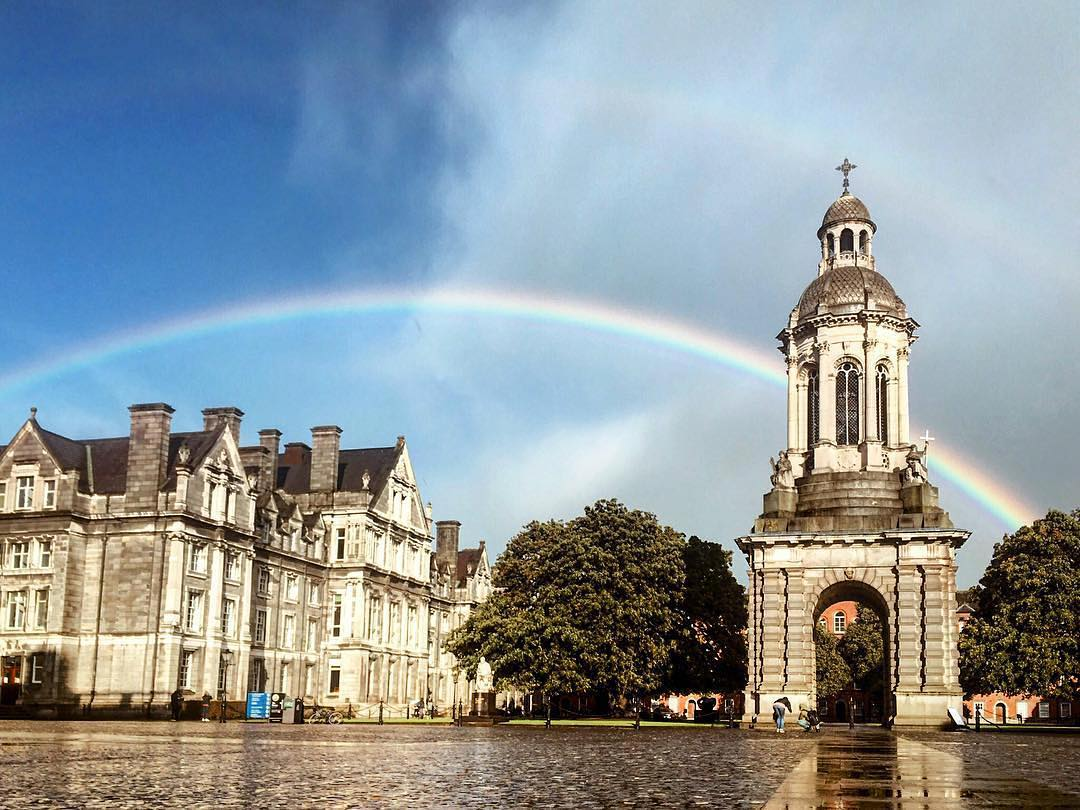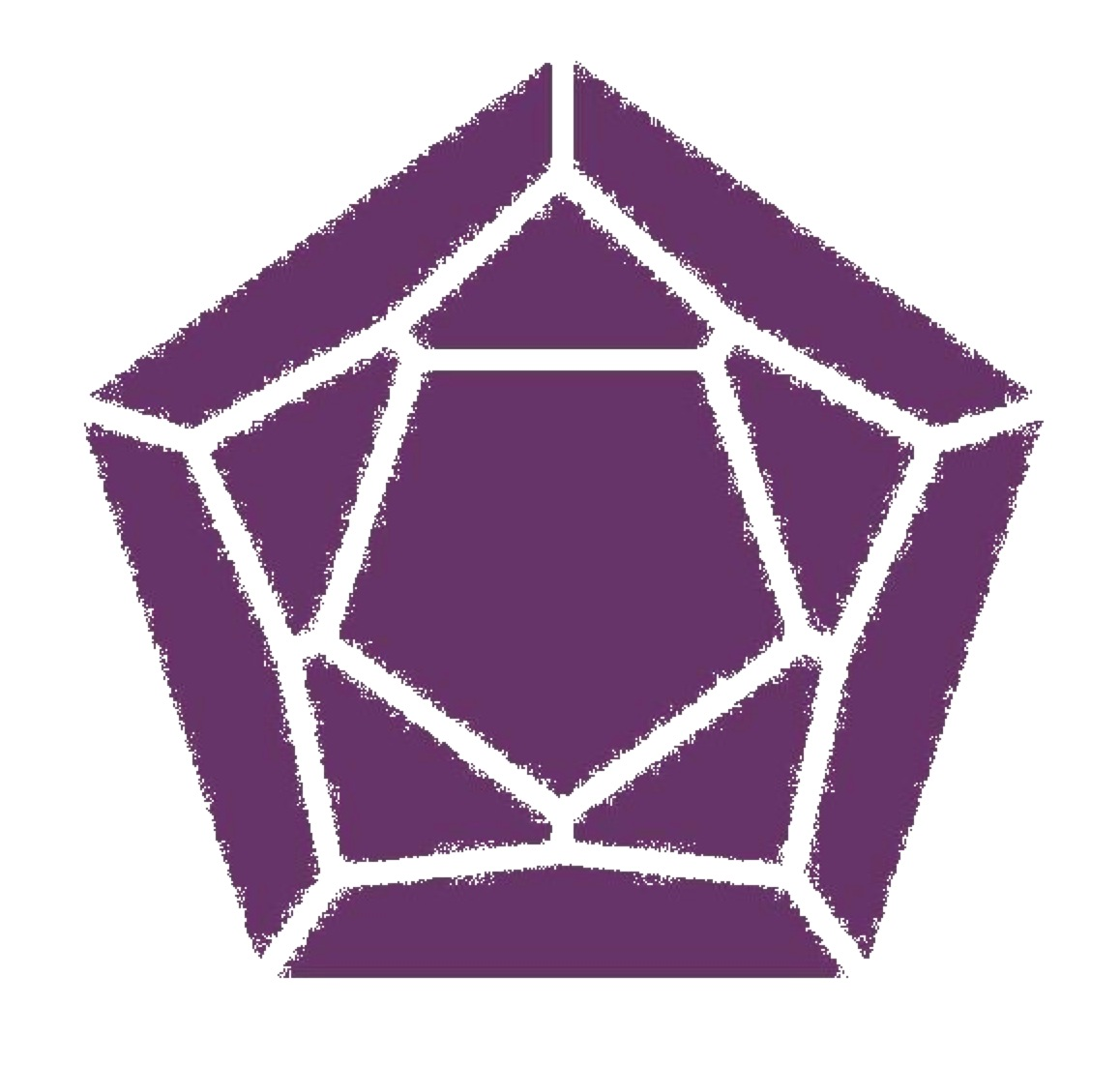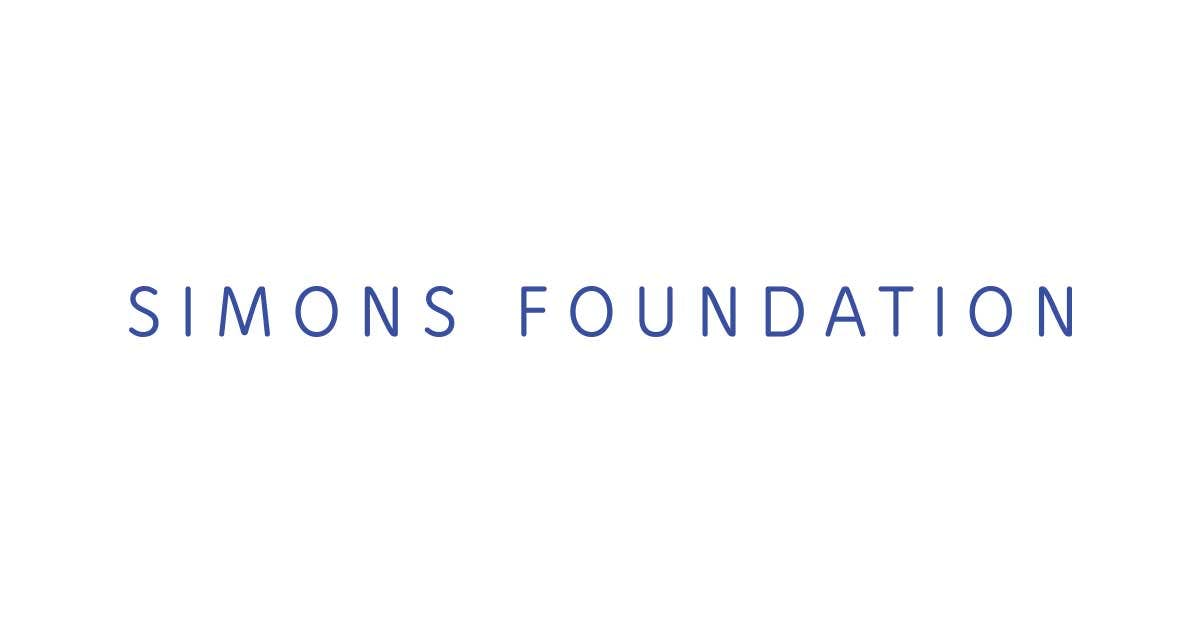# HMI Workshop # Hall algebras and related topics ### [Hamilton Mathematics Institute](http://www.tcd.ie/Hamilton/), [Trinity College Dublin](http://www.maths.tcd.ie/) #### November 27 - December 1, 2017 ##### The workshop is supported by [HMI](http://www.tcd.ie/Hamilton/) and [Simons Foundation](https://www.simonsfoundation.org) #### Speakers: * Ben Davison (University of Glasgow) * Markus Reineke (Bochum University) * Peter Samuelson (University of Edinburgh) * Matt Szczesny (Boston University) * Eoin Murphy (Sheffield University) #### Organiser: [Sergey Mozgovoy](http://www.maths.tcd.ie/~mozgovoy/) (HMI/TCD)

Registration:

Participant list. To register please write an email to

#### Location: * New Seminar Room, [Hamilton Building](https://www.maths.tcd.ie/about/map.php) * [Travel information](http://www.maths.tcd.ie/~dmc/travelinfo/)HMIDate Speaker Title Abstract Time
27.11.17 Matt Szczesny Hall algebras in the non-additive setting and combinatorial Hopf algebras Classically, Hall algebras arise by "counting short exact sequences" in finitary Abelian categories, such as the categories of quiver representations and categories of coherent sheaves on smooth projective varieties over $\mathbb{F}_q$. We will examine several examples of non-additive categories where this procedure still makes sense. The resulting Hall algebras are combinatorial Hopf algebras (or their duals), which include the Connes-Kreimer Hopf algebras of trees and Feynman graphs, the matroid-minor Hopf algebra, Hopf algebras constructed out of monoid representations on pointed sets, etc. 10am
Hall algebras over $\fun$ as limits of Hall algebras over $\mathbb{F}_q$ We will begin with a brief overview of the observations and analogies leading to the notion of $\fun$ - the "field of one element", and proceed to define the category of quiver representations over $\fun$. We'll see that the Hall algebra of this category recovers a degenerate version Ringel's construction of the positive half of the quantum group attached to the quiver. We move on to the next main source of Hall algebras - categories of coherent sheaves, but in the non-additive world. We discuss Deitmar's theory of monoid schemes and the corresponding category of coherent sheaves, using $\mathbb{P}^1_{\fun}$ as an explicit example. We'll see that Kapranov and Baumann-Kassel's result relating the Hall algebra of $\mathbb{P}^1_{\mathbb{F}_q}$ to a Borel in the quantum loop algebra of $\mathfrak{sl}_2$ holds at $q=1$. 11:30am
Towards the Hall algebra of projective surfaces over $\fun$ The examples of Hall algebras of quiver representations and coherent sheaves on $\mathbb{P^1}$ suggest that Hall algebra calculations over $\fun$ yield (potentially degenerate) specializations of the corresponding objects over $\mathbb{F}_q$. We sketch a description of the Hall algebra of $\mathbb{P}^2_{\fun}$ in terms of explicit combinatorial data such as skew shapes, simplicial partitions etc. in the hope that it may provide hints of what happens over $\mathbb{F}_q$. 3pm
28.11.17 Peter Samuelson Representations of the elliptic Hall algebra The elliptic Hall algebra of Burban and Schiffmann is an algebra depending on two parameters, q and t, and it is the "universal" Hall algebra of the category of coherent sheaves over an elliptic curve. In the t=q specialization, this algebra was shown to arise as the skein algebra of the torus [Morton, S], and as the trace of the q-Heisenberg category [Cautis, Lauda, Licata, S, Sussan]. In this talk we discuss these two realizations and show how they associate modules over the elliptic Hall algebra to 3-manifolds with torus boundary and to 2-representations of the q-Heisenberg category. 10am
The Fukaya category of a surface We discuss how the previous talk gives some hints that the Hall algebra of the Fukaya category of a surface may be related to the skein algebra of the surface, and to the elliptic Hall algebra when the surface is a torus. We then discuss the model of the Fukaya category of a surface with marked intervals on its boundary, due to Haiden, Katzarkov, and Kontsevich. We also recall a description of this category in terms of the path category of a quiver associated to the marked surface. 11:30am
The Hall algebra of Fukaya(S,M) Toen and [Xiao, Xu] defined the derived Hall algebra of a finitary triangulated category, and we discuss the derived Hall algebra of the Fukaya category of a marked surface [Cooper, S]. Based on previous work of Hernandez and Leclerc, we give a complete description of this algebra for the disk, and we give a conjectural description for surfaces with "enough marked intervals." 2:30pm
Eoin Murphy Integral forms and t=1 limits of Bridgeland-Hall algebras In the case of representations of ADE quivers we discuss a certain very natural integral form or subalgebra of the generic Bridgeland-Hall algebra. This subalgebra specializes at t=1 to a commutative Poisson-Hopf algebra of functions on a Poisson-Lie group. By computing the tangent Lie bialgebra one recovers the whole simple Lie algebra g from Bridgeland's construction. We show the integral form is isomorphic to an integral form of the quantized enveloping algebra due to De Concini and Procesi. At t=1 their integral form gives an algebra of functions on a Poisson-Lie group `dual' to one with Lie algebra g. We will also discuss the relationship with the t=1 limit of the integration map used in Donaldson-Thomas theory. This is work in progress for my PhD under Tom Bridgeland at the University of Sheffield. 4:00pm
29.11.17 Markus Reineke Chow-Hall algebras of quivers and Donaldson-Thomas invariants We identify the motivic Donaldson-Thomas invariants of a symmetric quiver with the Chow-Betti numbers of its moduli spaces of simple representations using structural results about its Chow-Hall algebra. This is joint work with H. Franzen. 10am
Gromov-Witten invariants and Donaldson-Thomas invariants of point configuration spaces We identify certain planar Gromov-Witten invariants computed by S. Fomin and G. Mikhalkin with the intersection Euler characteristic of moduli spaces of ordered point configurations in projective spaces. This is established by deriving a Kontsevich-type recursion for these Betti numbers from the defining recursion of motivic Donaldson-Thomas invariants for subspace quivers. This is joint work with T. Weist. 11:30am
Supports for linear degenerations of flag varieties We construct a flat family of so-called linear degenerations of flag varieties. We determine the supports of this familiy, controlling the variation of cohomology of the fibres, in combinatorial terms. This is achieved using a translation to a purely algebraic problem via G. Lusztig's Hall algebra approach to canonical bases of quantum groups. This is joint work with X. Fang. 2:30pm
30.11.17 Ben Davison The cohomological Hall algebra of a quiver with potential and its perverse filtration The cohomological Hall algebra associated to a quiver and potential is built from pulling back and pushing forward "critical cohomology", or the hypercohomology of vanishing cycles on the moduli stack of representations along a standard correspondence diagram. This algebra was introduced by Kontsevich and Soibelman as a "categorification" of the theory of Donaldson-Thomas partition functions. It turns out to be a very general fact that the map from the moduli stack to the coarse moduli space behaves very much like a proper map. Along with considering the maps induced by going the "wrong way" along the correspondence diagram, we finally arrive at the structure of a filtered localized bialgebra on the cohomological Hall algebra associated to a quiver with potential. 10am
The integrality theorem and quantum groups While the cohomological Hall algebra from the first talk looks like it is hard to tame - it is typically neither commutative or cocommutative, and the coproduct is only defined in a subtle "localized" way - after passing to the associated graded object the situation changes dramatically: the cohomological integrality theorem states that this bialgebra is in fact a free commutative algebra with a canonical PBW basis. That this basis is canonical is the extra ingredient that allows us to use this theorem to prove the integrality theorem for e.g. coherent sheaves on a Calabi-Yau 3-fold. 11:30am
Higher Kac-Moody Lie algebras and Yangians As suggested in the previous talk, the cohomological Hall algebra associated to a quiver with potential is a kind of quantum group. In addition, from considering just the first piece of the perverse filtration, we recover a Lie algebra. At least for certain choices of quiver with potential, this Lie algebra is the higher Kac-Moody algebra associated to some other quiver, in the sense that the usual KM algebra is the zeroth piece with respect to the cohomological grading. Conjecturally, the full algebra is the Maulik-Okounkov Yangian. 2:30pm

Timetable:

Time\Day Monday Tuesday Wednesday Thursday
9:40 Registration
10:00 Szczesny Samuelson Reineke Davison
11:00 Coffee Coffee Coffee Coffee
11:30 Szczesny Samuelson Reineke Davison
14:30 Samuelson Reineke Davison
15:00 Szczesny Coffee
16:00 Murphy
18:00 Workshop dinner